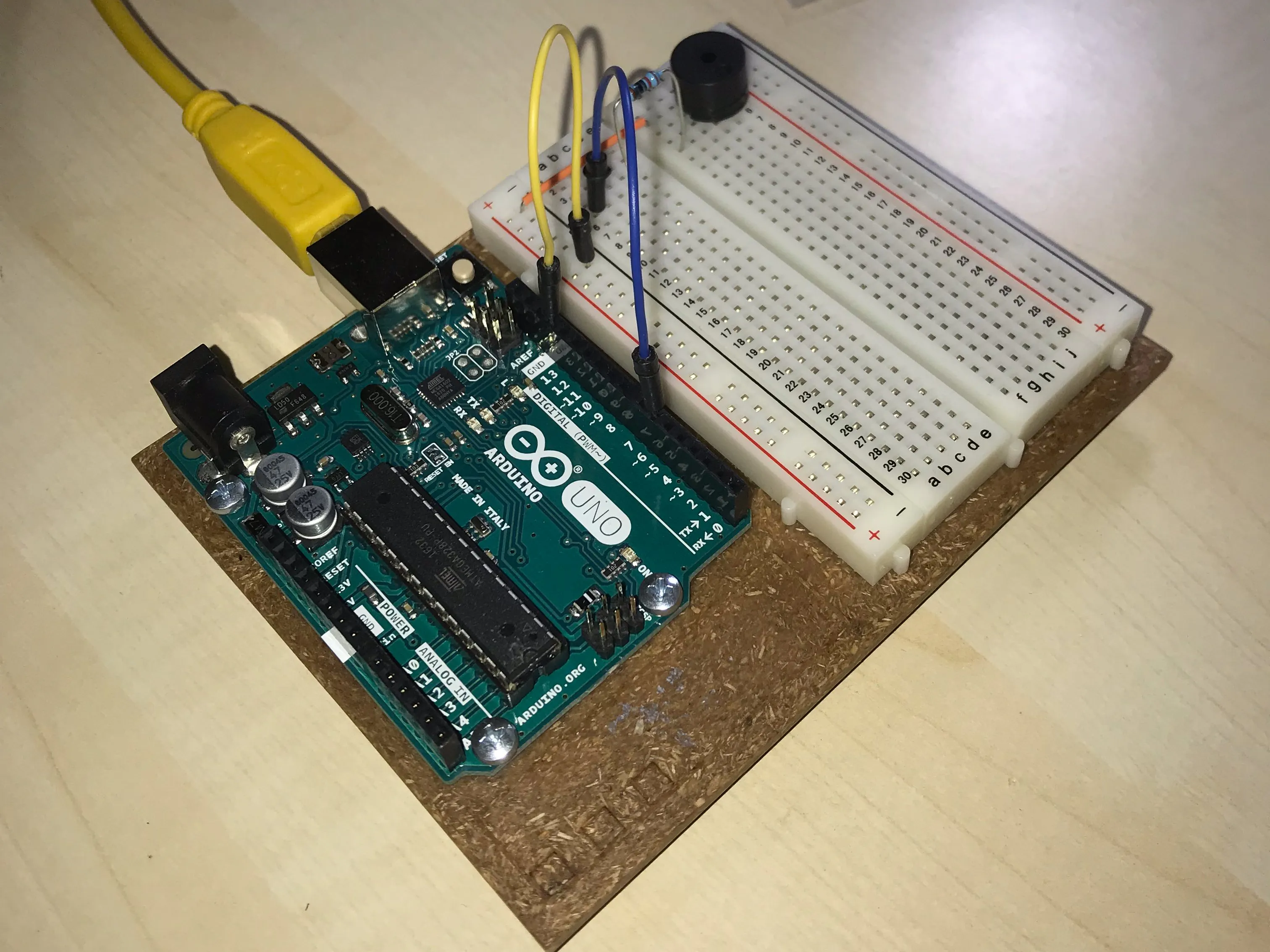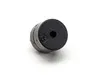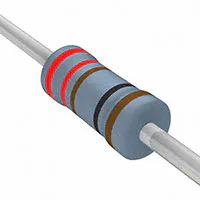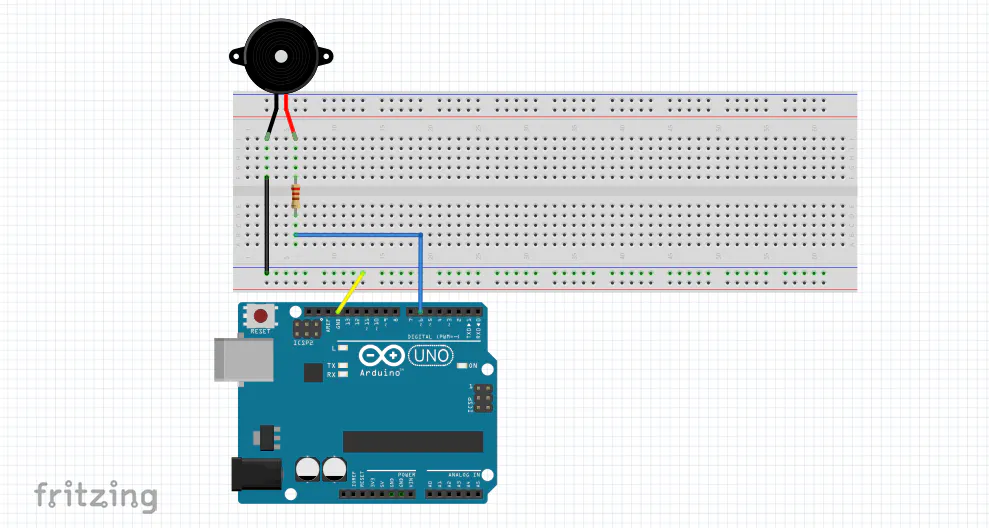# Keyboard V2.0

An update of the older version of the keyboard. Now it doesn't require switches on your breadboard, you can use your pc keyboard.

BeginnerWork in progress7 minutes11,504## Things used in this project

### Hardware componentsArduino UNO & Genuino UNO
×1Buzzer
×1Resistor 221 ohm
×1UTSOURCE Electronic Parts
×1

### Software apps and online servicesArduino IDE
 PuTTY

## Schematics

### Schematics for Arduino Keyboard 2.0

That's how you should wire your Arduino Keyboard 2.0## Code

### Code for Arduino Keyboard 2.0

C/C++
That's the code for your Arduino Keyboard 2.0
```#include "pitches.h"

int C[] = {N_C5};
int CS[] = {N_CS5};
int D[] = {N_D5};
int DS[] = {N_DS5};
int E[] = {N_E5};
int F[] = {N_F5};
int FS[] = {N_FS5};
int G[] = {N_G5};
int GS[] = {N_GS5};
int A[] = {N_A5};
int AS[] = {N_AS5};
int B[] = {N_B5};
int CC[] = {N_C6};
int duration(500);

void setup() {
pinMode(6, OUTPUT); //Digital Pin 6 is where you connect your Buzzer
Serial.begin(9600);
Serial.println("Welcome to the Virtual Keyboard!");
Serial.println("Notes: A-S-D-F-G-H-J");
Serial.println("#: Q-W-E-R-T-Y-U");
}

void loop() {
if (Serial.available()) {
if (ch == 'a') {
for (int Note = 0; Note < 1; Note++) {
tone(6, C[Note], duration);
}
}
if (ch == 'q') {
for (int Note = 0; Note < 1; Note++) {
tone(6, CS[Note], duration);
}
}
if (ch == 's') {
for (int Note = 0; Note < 1; Note++) {
tone(6, D[Note], duration);
}
}
if (ch == 'w') {
for (int Note = 0; Note < 1; Note++) {
tone(6, DS[Note], duration);
}
}
if (ch == 'd') {
for (int Note = 0; Note < 1; Note++) {
tone(6, E[Note], duration);
}
}
if (ch == 'f') {
for (int Note = 0; Note < 1; Note++) {
tone(6, F[Note], duration);
}
}
if (ch == 'r') {
for (int Note = 0; Note < 1; Note++) {
tone(6, FS[Note], duration);
}
}
if (ch == 'g') {
for (int Note = 0; Note < 1; Note++) {
tone(6, G[Note], duration);
}
}
if (ch == 't') {
for (int Note = 0; Note < 1; Note++) {
tone(6, GS[Note], duration);
}
}
if (ch == 'h') {
for (int Note = 0; Note < 1; Note++) {
tone(6, A[Note], duration);
}
}
if (ch == 'y') {
for (int Note = 0; Note < 1; Note++) {
tone(6, AS[Note], duration);
}
}
if (ch == 'j') {
for (int Note = 0; Note < 1; Note++) {
tone(6, B[Note], duration);
}
}
if (ch == 'u') {
for (int Note = 0; Note < 1; Note++) {
tone(6, CC[Note], duration);
}
}
}
}
```

## Credits

### Gabriele Scordamaglia

6 projects • 36 followers
21 YO, Electronics Engineering student in Italy.